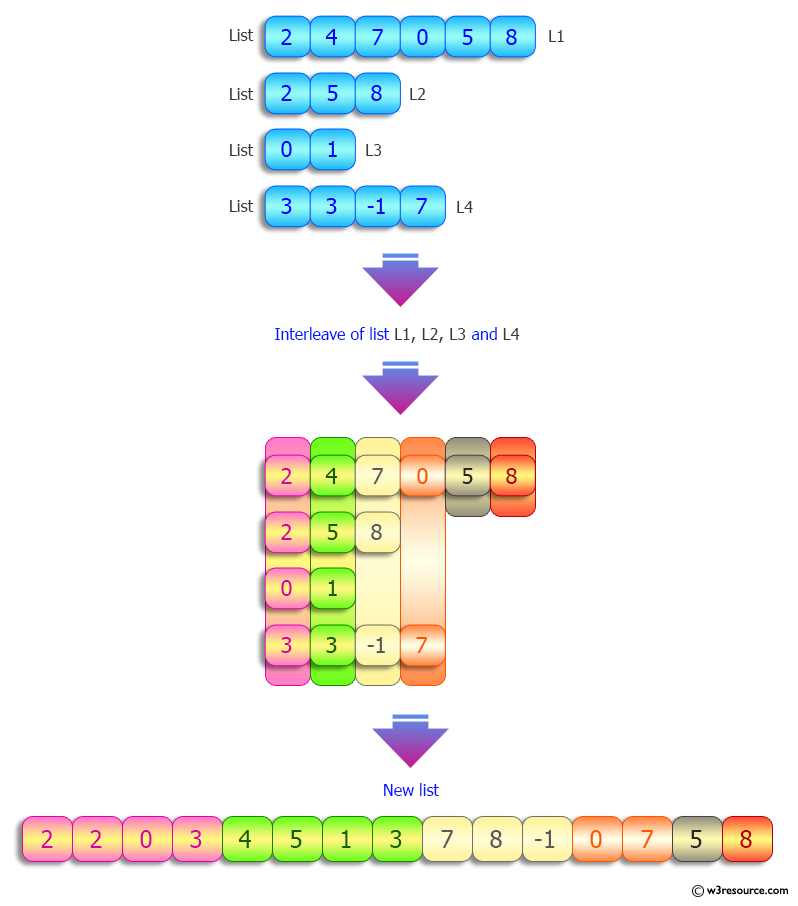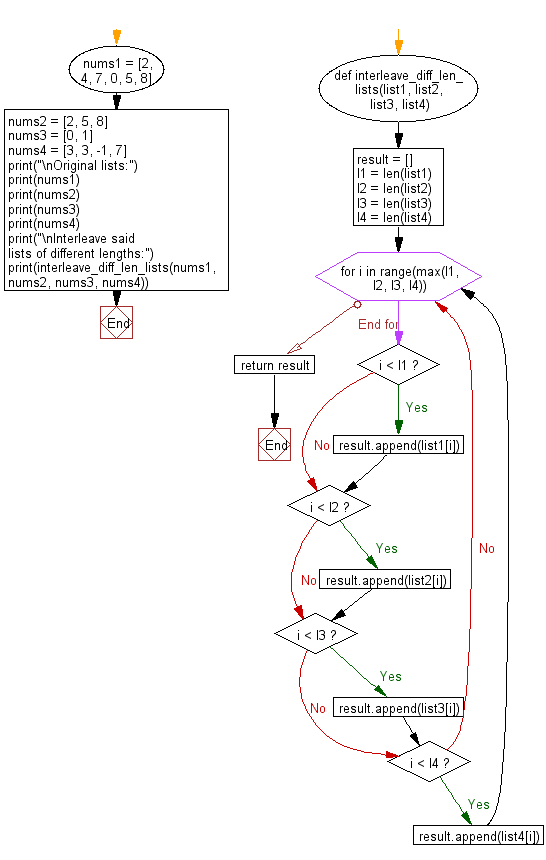﻿ Python: Interleave multiple lists of different lengths - w3resource# Python: Interleave multiple lists of different lengths

## Python List: Exercise - 157 with Solution

Write a Python program to interleave multiple given lists of different lengths.

Sample Solution:

Python Code:

``````def interleave_diff_len_lists(list1, list2, list3, list4):
result = []
l1 = len(list1)
l2 = len(list2)
l3 = len(list3)
l4 = len(list4)

for i in range(max(l1, l2, l3, l4)):
if i < l1:
result.append(list1[i])
if i < l2:
result.append(list2[i])
if i < l3:
result.append(list3[i])
if i < l4:
result.append(list4[i])
return result

nums1 = [2, 4, 7, 0, 5, 8]
nums2 = [2, 5, 8]
nums3 = [0, 1]
nums4 = [3, 3, -1, 7]

print("\nOriginal lists:")
print(nums1)
print(nums2)
print(nums3)
print(nums4)

print("\nInterleave said lists of different lengths:")
print(interleave_diff_len_lists(nums1, nums2, nums3, nums4))
```
```

Sample Output:

```Original lists:
[2, 4, 7, 0, 5, 8]
[2, 5, 8]
[0, 1]
[3, 3, -1, 7]

Interleave said lists of different lengths:
[2, 2, 0, 3, 4, 5, 1, 3, 7, 8, -1, 0, 7, 5, 8]
```

Pictorial Presentation:Flowchart:## Visualize Python code execution:

The following tool visualize what the computer is doing step-by-step as it executes the said program:

Python Code Editor:

Have another way to solve this solution? Contribute your code (and comments) through Disqus.

What is the difficulty level of this exercise?

Test your Python skills with w3resource's quiz

﻿

## Python: Tips of the Day

```print(2_000_000)
```2000000